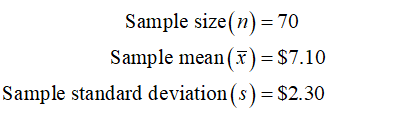# Sam is the manager of a used clothing store.  He wants to estimate the average amount a customer spends per visit.  A random sample of 70 customers’ receipts gave a mean of = \$7.10 with a standard deviation s = \$2.30.  Find a 99% confidence interval for the average amount spent by all customers. Interpret your findings.

Question
7 views

Sam is the manager of a used clothing store.  He wants to estimate the average amount a customer spends per visit.  A random sample of 70 customers’ receipts gave a mean of = \$7.10 with a standard deviation s = \$2.30.  Find a 99% confidence interval for the average amount spent by all customers. Interpret your findings.

check_circle

Step 1

From the provided information,The population standard deviation is not known therefore t distribution will be used to obtain the confidence interval.

The degree of freedom = n – 1 = 70 – 1 = 69

99% confidence level means α = 1 – 0.99 = 0.01

The critical value of t at 0.01 level of significance with 69 degre...

### Want to see the full answer?

See Solution

#### Want to see this answer and more?

Solutions are written by subject experts who are available 24/7. Questions are typically answered within 1 hour.*

See Solution
*Response times may vary by subject and question.
Tagged in